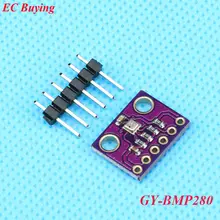Home Raspberry PI Raspberry Piand BMP280 barometric pressure sensor Java example

# Raspberry Piand BMP280 barometric pressure sensor Java example

BMP280 is an absolute barometric pressure sensor especially designed for mobile applications. The sensor module is housed in an extremely compact package. Its small dimensions and its low power consumption allow for the implementation in battery powered devices such as mobile phones, GPS modules or watches.

As its predecessor BMP180, BMP280 is based on Bosch’s proven Piezo-resistive pressure sensor technology featuring high accuracy and linearity as well as long term stability and high EMC robustness. Numerous device operation options offer highest flexibility to optimize the device regarding power consumption, resolution and filter performance. A tested set of default settings for example use case is provided to the developer in order to make design-in as easy as possible.Applications

– Indoor navigation (floor detection, elevator detection)

– Outdoor navigation, leisure and sports applications

– Weather forecast

– Health care applications (e.g. spirometry)

– Vertical velocity indication (e.g. rise/sink speed)

Parameter Technical data
Operation range (full accuracy) Pressure: 300…1100 hPa
Temperature: -40…85°C
Absolute accuracy
(Temp. @ 0…+65°C)
~ ±1 hPa
Relative accuracy
p = 700…900hPa
(Temp. @ +25…+40°C)
± 0.12 hPa (typical)
equivalent to ±1 m
Average current consumption (1 Hz data refresh rate) 2.74 μA, typical
(ultra-low power mode)
Average current consumption in sleep mode 0.1 μA
Average measurement time 5.5 msec
(ultra-low power preset)
Supply voltage VDDIO 1.2 … 3.6 V
Supply voltage VDD 1.71 … 3.6 V
Resolution of data Pressure: 0.01 hPa ( < 10 cm)
Temperature: 0.01° C
Temperature coefficient offset
(+25°…+40°C @900hPa)
± 0.12 hPa (typical)
equivalent to ±1 m
Interface I²C and SPI

### Layout

This is a layout diagram using an adafruit part, my module had clearly marked SDA and SCL connections

### Code

You need to install Pi4J – http://pi4j.com/install.html

[codesyntax lang=”java”]

```// Distributed with a free-will license.
// Use it any way you want, profit or free, provided it fits in the licenses of its associated works.
// BMP280
// This code is designed to work with the BMP280_I2CS I2C Mini Module available from ControlEverything.com.
// https://www.controleverything.com/content/Barometer?sku=BMP280_I2CSs#tabs-0-product_tabset-2

import com.pi4j.io.i2c.I2CBus;
import com.pi4j.io.i2c.I2CDevice;
import com.pi4j.io.i2c.I2CFactory;
import java.io.IOException;

public class BMP280
{
public static void main(String args[]) throws Exception
{
// Create I2C bus
I2CBus bus = I2CFactory.getInstance(I2CBus.BUS_1);
// Get I2C device, BMP280 I2C address is 0x76(108)
I2CDevice device = bus.getDevice(0x76);

byte[] b1 = new byte;

// Convert the data
// temp coefficents
int dig_T1 = (b1 & 0xFF) + ((b1 & 0xFF) * 256);
int dig_T2 = (b1 & 0xFF) + ((b1 & 0xFF) * 256);
if(dig_T2 > 32767)
{
dig_T2 -= 65536;
}
int dig_T3 = (b1 & 0xFF) + ((b1 & 0xFF) * 256);
if(dig_T3 > 32767)
{
dig_T3 -= 65536;
}

// pressure coefficents
int dig_P1 = (b1 & 0xFF) + ((b1 & 0xFF) * 256);
int dig_P2 = (b1 & 0xFF) + ((b1 & 0xFF) * 256);
if(dig_P2 > 32767)
{
dig_P2 -= 65536;
}
int dig_P3 = (b1 & 0xFF) + ((b1 & 0xFF) * 256);
if(dig_P3 > 32767)
{
dig_P3 -= 65536;
}
int dig_P4 = (b1 & 0xFF) + ((b1 & 0xFF) * 256);
if(dig_P4 > 32767)
{
dig_P4 -= 65536;
}
int dig_P5 = (b1 & 0xFF) + ((b1 & 0xFF) * 256);
if(dig_P5 > 32767)
{
dig_P5 -= 65536;
}
int dig_P6 = (b1 & 0xFF) + ((b1 & 0xFF) * 256);
if(dig_P6 > 32767)
{
dig_P6 -= 65536;
}
int dig_P7 = (b1 & 0xFF) + ((b1 & 0xFF) * 256);
if(dig_P7 > 32767)
{
dig_P7 -= 65536;
}
int dig_P8 = (b1 & 0xFF) + ((b1 & 0xFF) * 256);
if(dig_P8 > 32767)
{
dig_P8 -= 65536;
}
int dig_P9 = (b1 & 0xFF) + ((b1 & 0xFF) * 256);
if(dig_P9 > 32767)
{
dig_P9 -= 65536;
}
// Select control measurement register
// Normal mode, temp and pressure over sampling rate = 1
device.write(0xF4 , (byte)0x27);
// Select config register
// Stand_by time = 1000 ms
device.write(0xF5 , (byte)0xA0);

// pressure msb1, pressure msb, pressure lsb, temp msb1, temp msb, temp lsb, humidity lsb, humidity msb
byte[] data = new byte;

// Convert pressure and temperature data to 19-bits
long adc_p = (((long)(data & 0xFF) * 65536) + ((long)(data & 0xFF) * 256) + (long)(data & 0xF0)) / 16;
long adc_t = (((long)(data & 0xFF) * 65536) + ((long)(data & 0xFF) * 256) + (long)(data & 0xF0)) / 16;

// Temperature offset calculations
double var1 = (((double)adc_t) / 16384.0 - ((double)dig_T1) / 1024.0) * ((double)dig_T2);
double var2 = ((((double)adc_t) / 131072.0 - ((double)dig_T1) / 8192.0) *
double t_fine = (long)(var1 + var2);
double cTemp = (var1 + var2) / 5120.0;
double fTemp = cTemp * 1.8 + 32;

// Pressure offset calculations
var1 = ((double)t_fine / 2.0) - 64000.0;
var2 = var1 * var1 * ((double)dig_P6) / 32768.0;
var2 = var2 + var1 * ((double)dig_P5) * 2.0;
var2 = (var2 / 4.0) + (((double)dig_P4) * 65536.0);
var1 = (((double) dig_P3) * var1 * var1 / 524288.0 + ((double) dig_P2) * var1) / 524288.0;
var1 = (1.0 + var1 / 32768.0) * ((double)dig_P1);
double p = 1048576.0 - (double)adc_p;
p = (p - (var2 / 4096.0)) * 6250.0 / var1;
var1 = ((double) dig_P9) * p * p / 2147483648.0;
var2 = p * ((double) dig_P8) / 32768.0;
double pressure = (p + (var1 + var2 + ((double)dig_P7)) / 16.0) / 100;

// Output data to screen
System.out.printf("Pressure : %.2f hPa %n", pressure);
System.out.printf("Temperature in Celsius : %.2f C %n", cTemp);
System.out.printf("Temperature in Fahrenheit : %.2f F %n", fTemp);
}
}```

[/codesyntax]

Open the terminal

Compile the java program.

`\$> sudo pi4j BMP280.java`

Run the java program.

`\$> sudo pi4j BMP280`

### Output